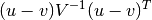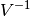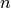# scipy.spatial.distance.mahalanobis¶

scipy.spatial.distance.mahalanobis(u, v, VI)

Computes the Mahalanobis distance between two n-vectors u and v, which is defiend aswhere VI is the inverse covariance matrix.

Parameters : u : ndarray An-dimensional vector. v : ndarray An-dimensional vector. d : double The Mahalanobis distance between vectors u and v.

#### Previous topic

scipy.spatial.distance.kulsinski

#### Next topic

scipy.spatial.distance.matching